# When Will All Cars be Electric?

L. David Roper, http://www.roperld.com/personal/roperldavid.htm
09-Dec-2018

## Introduction

This is an attempt to estimate how fast electric cars will replace gasoline and diesel cars. It will use the data for cars for several past years. Pickups and trucks have not been consider in this analysis.

Definition of "Electric Car" or "Plug-In Car":
Any car with a battery that provides part or all of the energy for movement of the car and for which the battery can be charged from the grid or solar panels. This includes plug-in hybrid cars (PHEVs) and battery-electric cars (BEVs). The data so far indicate that about half of electric cars put on the road in the U.S. are PHEV and half are BEVs.

## Number of All Cars

The number of cars worldwide for different years are given in https://en.wikipedia.org/wiki/Motor_vehicle and in the follow graph: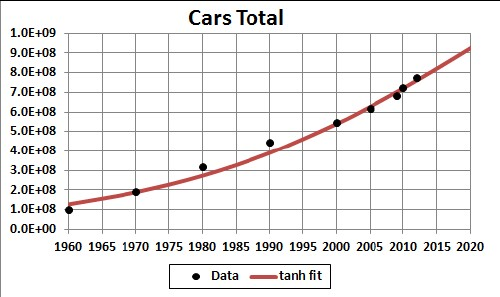The hyperbolic-tangent fit is only to data from year 2000. The tanh time constant is ~40 years, which corresponds to an exponential time constant of ~20 years.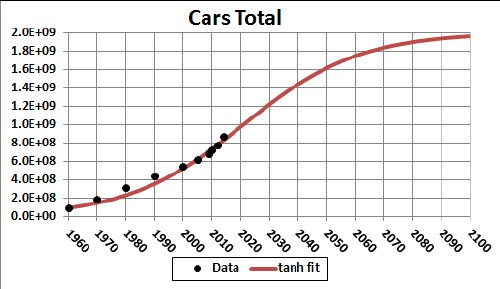The current ratio of cars to population is about 0.1. It appears that the final asymptotic population of the world will be about 10 billion , assuming that the population does not collapse. I assume that the ratio of cars to population will asymptotically be about 0.2; so the asymptotic number of cars would be 2 billion. The red curve in the graph above is a hyperbolic-tangent fit to the data from 2000 on with a 2-billion asymptote: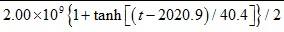. This equation has the same exponential time constant, 47.2 years, approaching the asymptote as the rising time constant; below that will be revised.

I choose a small car/population ratio of 0.2 for the following reasons:

• Migration into cities, where the car/population ratio is small, is continuing.
• Mass transportation is increasing.
• Car sharing is increasing.
• Crude oil extraction is near its peak.
• Increasing global warming will necessitate not burning fossil fuels.
Go to top.

## Number of Electric Cars

The number of electric cars worldwide for different years are given in http://www.pluginamerica.org/drivers-seat/plug-electric-vehicle-sales-pace-double-first-five-years-plugless-hybrid-sales and in the following graph: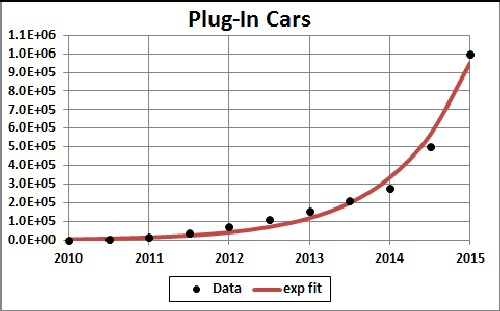The red curve is an exponential fit with time constant of 0.96 years.

If one assumes that the number of electric cars follows a hyperbolic-tangent curve with a 2-billion asymptote, the curve will be: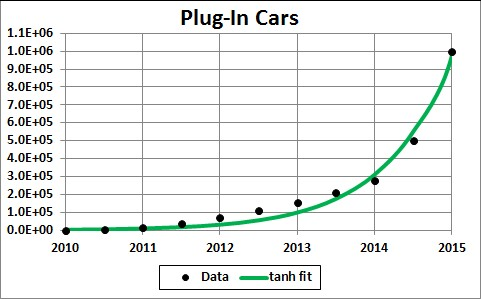The green curve is a hyperbolic-tangent fit to the data: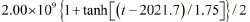The hyperbolic-tangent time constant is twice the exponential time constant by the mathematics of the two equations. The fit curve approaches a 2-billion asymptote, as shown by the red curve below: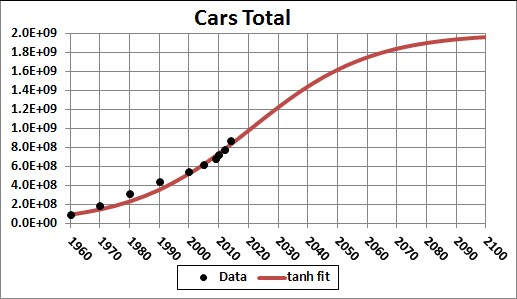Go to top.

## Revision of Number of Electric Cars

Of course, the green curve, which is for electric cars, cannot exceed the red curve for all cars; instead, it must merge into the red curve. An equation to achieve that merge is the Verhulst function: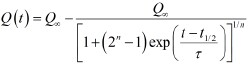This function is used to fit the plug-ins data such that the asymptote is 2 x 109 cars eventually and smoothly joins the red total-cars curve.

The Verhulst fit to the plug-ins data is: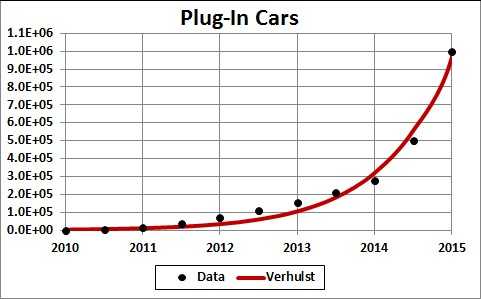The parameters for the Verhulst fit are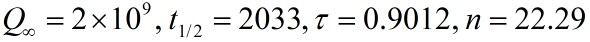Thus, the initial exponential time constant is 0.9012 years and the final exponential time constant is 22.29 x 0.9012 = 20.09 years.

The smoothing of plug-in cars into the total cars red curve is given by the green curve here:Note that the term "plug-in car" is any car with a battery that can be charged by plugging it into a power source, whether or not it also has a liquid-fuel engine or is a BEV.

Of course, it may turn out that the current exponential rise in electric cars will not continue so fast or it may be faster.

This compares well with the amount of crude oil left to be extracted for the world: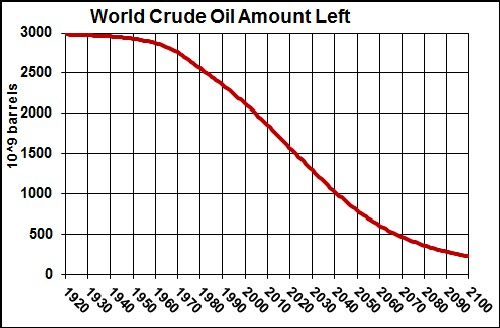The transition from gasoline/diesel cars to electric cars will begin to be rapid when the amount of crude oil left is down to about half of the total extraction at ~2020. The transition to electric cars will be much faster than the decline in crude-oil extraction partly because electric cars are much better that gasoline/diesel cars in almost every aspect. The recent fast exponential rise of electric cars attests to that.

## Conclusion

We can expect a rapid transition from gasoline/diesel cars to electric cars between 2020 and 2030. First many of the electric cars will be plug-in hybrid cars (PHEVs), but eventually almost all will be pure battery-electric cars (BEVs).

Pickups and trucks have not been consider is this analysis.

Go to top.

http://www.roperld.com/personal/InterdisciplinaryStudies.htm